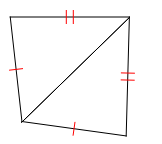Proving Triangles Congruent
3 years ago
nwancell
Save
Edit
Host a game
Live GameLive
Homework
Solo Practice
Practice14 QuestionsShow answers
• Question 1
120 secondsQ. Classify the triangle by its sides
equilateral
isosceles
scalene
• Question 2
120 secondsQ. Classify the triangle by its angles
equiangular
acute
right
obtuse
• Question 3
120 seconds
Q. A triangle has angle measures of
m<A = 2x +10
m<B = 5x - 3
m<C = 3x - 7
Find x.
85
36.6
62.3
18
• Question 4
120 secondsQ. What is the measure of the missing angle?
20
130
30
50
• Question 5
120 secondsQ. What is the measure of the missing angle?
20
47
29
16
• Question 6
120 secondsQ. Can the triangles be proven congruent?  If so, how?
ASA
AAS
SAS
Cannot be proven congruent
• Question 7
120 secondsQ. Can the triangles be proven congruent?  If so, how?
ASA
AAS
SAS
Cannot be proven congruent
• Question 8
120 secondsQ. Can the triangles be proven congruent?  If so, how?
ASA
AAS
SAS
Cannot be proven congruent
• Question 9
120 secondsQ. Can the triangles be proven congruent?  If so, how?
ASA
AAS
SAS
Cannot be proven congruent
• Question 10
120 secondsQ. Can the triangles be proven congruent?  If so, how?
ASA
AAS
SAS
Cannot be proven congruent
• Question 11
120 secondsQ. Can the triangles be proven congruent?  If so, how?
ASA
Cannot be proven congruent
SAS
SSS
• Question 12
120 secondsQ. What missing piece of information is needed to prove the triangles congruent using SAS?
∠F≅∠Q
∠G≅∠R
∠H≅∠S
FH = QS
• Question 13
120 secondsQ. What missing piece of information is needed to prove the triangles congruent using ASA?
GC = CD
CE = CD
∠GCE≅∠BCE
BD = GE
• Question 14
120 secondsQ. What missing piece of information is needed to prove the triangles congruent using SAS?